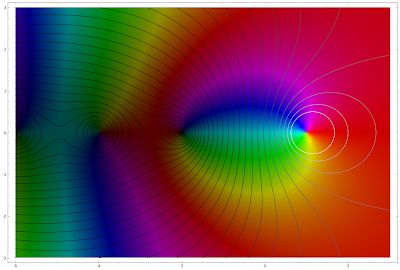## Wednesday, March 23, 2011

### Sci-fi history in a painting

This is pretty fantastic - Ward Shelley's "The History of Sci­ence Fiction":(The crop above is just the tip of the iceberg.)

## Monday, March 21, 2011

### Plotting the zeta function

I lectured a tiny bit on the Riemann zeta function for the first time in my complex analysis course, which inspired me to make the following plot. Colors are the argument of the Riemann zeta function, brightness is proportional to magnitude. The default brightness map gives very low contrast, so I modified the magnitudes. To help with seeing the magnitudes a contour map with exponentially spaced contours is overlaid.

def xy_to_zeta_size(x,y):    return abs(zeta(N(x+I*y)))cvals = [e^i for i in srange(-7,1,.25)]cp = contour_plot(xy_to_zeta_size,(-6,3),(-3,3),contours = cvals, fill=False, plot_points = 201)rzeta(z) = zeta(z)/norm(zeta(z))^(.25)rzf = fast_callable(rzeta,domain=CDF)cparg = complex_plot(rzf,(-6,3),(-3,3))show(cparg+cp,figsize=[18,12])